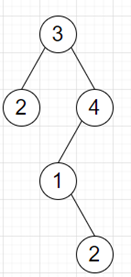# Program to count number of paths whose sum is k in python

Suppose we have a binary tree and another value k, we have to find the number of unique node to sub child paths are there which sums to k.

So, if the input is likeand k = 5, then the output will be 2, as the paths are [2, 3] and [1, 4]

To solve this, we will follow these steps −

• count := a map initially holds value 1 for key 0
• ans := 0, prefix := 0
• Define a function dfs() . This will take node
• if node is not null, then
• prefix := prefix + value of node
• ans := ans + (count[prefix - target], if this is not available, it will be 0)
• count[prefix] := count[prefix] + 1
• dfs(left of node)
• dfs(right of node)
• count[prefix] := count[prefix] - 1
• prefix := prefix - value of node
• From the main method, do the following
• dfs(root)
• return ans

Let us see the following implementation to get better understanding −

## Example

Live Demo

from collections import Counter
class TreeNode:
def __init__(self, data, left = None, right = None):
self.val = data
self.left = left
self.right = right

class Solution:
def solve(self, root, target):
count = Counter()
ans = prefix = 0

def dfs(node):
nonlocal ans, prefix
if node:
prefix += node.val
ans += count[prefix - target]
count[prefix] += 1
dfs(node.left)
dfs(node.right)

count[prefix] -= 1
prefix -= node.val
dfs(root)
return ans

ob = Solution()
root = TreeNode(3)
root.left = TreeNode(2)
root.right = TreeNode(4)
root.right.left = TreeNode(1)
root.right.left.right = TreeNode(2)
k = 5
print(ob.solve(root, k))

## Input

root = TreeNode(3)
root.left = TreeNode(2)
root.right = TreeNode(4)
root.right.left = TreeNode(1)
root.right.left.right = TreeNode(2)
5

## Output

2